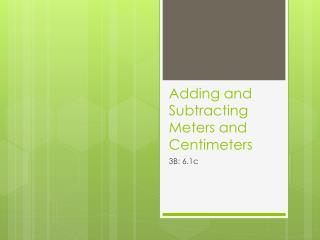DownloadDownload PresentationAdding and Subtracting Meters and Centimeters

# Adding and Subtracting Meters and Centimeters

Télécharger la présentation## Adding and Subtracting Meters and Centimeters

- - - - - - - - - - - - - - - - - - - - - - - - - - - E N D - - - - - - - - - - - - - - - - - - - - - - - - - - -
##### Presentation Transcript

1. Adding m & cm with no renaming 2 85 • 2 m 60 cm + 25 cm = _____ m _____ cm • How do you get the answer? • Since there are no meters in front of 25 cm, just add the centimeters.

2. Adding m & cm with renaming 3 35 • 2 m 60 cm + 75 cm = _____ m _____ cm • You can still add the centimeters since there are no meters in front of the 75 cm. • 60 cm + 75 cm = 135 cm • Can you leave the 135 cm as it is? • Convert it into m and cm. • 1 m 35 cm + 75 cm 2 m 135 cm = 2 m 60 cm 3 m 35 cm

3. Another way • You could also make 100 with either 60 cm or 75 cm. 75 cm 2 m 60 cm + 35 cm 25 cm 2 m 35 cm 1 m 3 m 35 cm + =

4. Another way • Now let’s make 100 with the 60. How much do we need from the 75? • How much is left? 75 cm 2 m 60 cm + 40cm 35 cm 2 m 3 m 35 cm 1 m 35 cm + =

5. One more strategy… • You can add the numbers up vertically. • First, convert into cm. • Add. • Convert back to m and cm. 2m 60 cm 260 cm + 75 cm + 75 cm 3m 35 cm 335 cm

6. Adding both meters and centimeters • 2 m 65 cm + 3 m 20 cm = _____ m _____cm • Use mental calculation to add the meters. • Then add the centimeters. 2 m 65 cm + 3 m 20 cm 5 m 65 cm + 20 cm 5 m 85 cm

7. Adding both meters and centimeters • Try this one… • 2 m 65 cm + 3 m 40 cm = _____ m _____cm • Add the meters. • Then add the centimeters and rename. 2 m 65 cm + 3 m 40 cm 5 m 65 cm + 40 cm 5 m 105 cm 6 m 5 cm

8. Use vertical adding, if you prefer • First, convert into cm. • Add. • Convert back to m and cm. 2 m 65 cm 265 cm +3 m 40 cm + 340 cm 6 m 5 cm 605 cm

9. Subtracting both meters and centimeters 5 5 • 5 m 30 cm – 25 cm = _____m _____ cm • To find the answer, subtract 25 cm from 30 cm. There are no meters to take away so the 5 m stays the same.

10. Subtracting both meters and centimeters 4 55 • 5 m 30 cm – 75 cm = _____m _____ cm • Take 100 cm from the 5 meters. What’s left? • Subtract the 75 from the 100. • Add the remaining cm together. 75 cm = 5 m 30 cm – = 4 m 30 cm + 25 cm 4 m 30 cm 100 cm 75 cm – = 4 m 55 cm

11. Other strategies • Is there another way to solve the problem 5 m 30 cm – 75 cm? • You can rename 5 m 30 cm. • Then subtract. 5 m 30 cm – 75 cm = 4 m 130 cm – 75 cm = 4 m 55 cm

12. Use vertical subtracting, if you prefer • First, convert into cm. • Subtract. • Convert back to m and cm. 5 m 30 cm 530 cm – 75 cm – 75 cm 4 m 55 cm 455 cm

13. Subtracting when you don’t have to rename • 5 m 30 cm – 2 m 25 cm = ____ m _____ cm • How should we solve this? • Subtract meters first. • Then subtract centimeters. – 2 m – 25 cm 5 m 30 cm 3 m 30 cm 3 m 5 cm

14. RENAME CONVERT • How do we find the difference between 5 m 30 cm and 2 m 75 cm? • Let’s look at three subtraction strategies… 5 m 30 cm – 2 m 75 cm = 5 m 30 cm 530 cm – 2 m 75 cm – 275 cm 3 m 30 cm – 75 cm = 255 cm 2 m 55 cm 2 m 130 cm – 75 cm = 2 m 55 cm MAKE 100 2 m 75 cm = 5 m 30 cm – 3 m 30 cm – 75 cm = = 2 m 30 cm + 25 cm 2 m 30 cm 100 cm 75 cm – = 2 m 55 cm

15. Now you try. • Textbook page 12 • Tasks 14-15 • 14 a) 5m 25cm b) 1m 55cm • 15 a) 9m 32cm b) 3m 79cm • c) 8m 30cm d) 6m 46cm • e) 3m 20cm f) 9m 1cm • g) 6m 15cm h) 5m 24cm • i) 4m 55cm j) 3m 95cm • k) 3m 55cm l) 2m 30cm

16. Extra practice and/or Homework • WB pages 9-10 • EP 113-114 • Review 1B